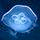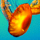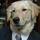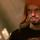## General Question# Why do derivatives appear so often in physics and other high-level math-based sciences?

Asked by quarkquarkquark (1695) May 7th, 2009

I see derivatives in relativistic physics all the time, and all I remember from calculus is that their practical use is finding the area under a curve. Why do derivatives seem to be everywhere?

Observing members: 0Composing members: 0Derivatives measure the rate of change of any variable, they can be used whenever there is a value that is changing. Area under a curve is just one application.

iamart (18)“Great Answer” (3) Flag as…Response moderatedThe derivatives are preparing an invasion. You didn’t hear this from me.

Fyrius (14555)“Great Answer” (1) Flag as…Incidentally, integrals find the area under a curve; derivatives find the instantaneous rate of change.
The reason these tools are everywhere is that there are very few instances in which values are always the same at every point in time and space. They could be changing as a function of time, they could be changing as a function of their distance from a point, they could be changing as a function of themselves, and if you want to find the accumulated effect of all of these values, you have to use an integral; that’s what an integral is, a tool for finding the aggregate value of an infinity of infinitesimal values. Of course, wherever you have an integral, you have a derivative. So if you want to find how fast something is changing as you change something else, you use a derivative, and if you want to find the value of something that has been changing at the rate described by that derivative, you use its integral. Pretty general, right? That’s why they’re everywhere.

Jayne (6756)“Great Answer” (8) Flag as…That was a very clear explanation; thank you!

quarkquarkquark (1695)“Great Answer” (0) Flag as…or